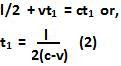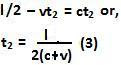## Monday, November 16, 2009

### Irodov Problem 1.351This is a problem that illustrates the classic breakdown of simultaneity in relativistic mechanics. Before we get into solving the problem let us first try to understand what is happening.

There are three important things to understand,
i) light speed as seen in any reference frame is the same and equal to c.
ii) an observer feels that an event occurred when the event reaches him and events travel at the speed of light.
iii) If the location and time of two events are equal in one frame then the location and time of the two events in some other frame will also be the equal. In other words if two cars collide in one frame (they occupy equal space and time) then the cars will collide in all other frames (they will occupy equal space and time - note that values of these may differ in different frames).

For simplicity's sake consider an observer O in the mid-point between the two particles, moving at the same velocity as the particles (note that the placement of the observer is not crucial to solving the problem, it only simplifies it - I shall explain this in more detail later in the solution). Consider another observer O' who is located in the laboratory reference frame (frame K), and as seen by O happened to be at the same position as him when the particles decayed (again this location of O' has been chosen for simplicity, and this does not alter the final answer).

Events as seen from the particle frame of reference:
O sees that both the particles decayed at the same time. This means that the event of particle decay from both particles reached the observer at the same time. Imagine that each decay was accompanied by a brilliant flash - then both the flashes reached the observer at exactly the same time. Since O is at the mid-point between the particles and the particles are stationary as seen by O, O deduces that the particles decayed at exactly the same time.

Meanwhile as seen by O, an observer O' who is in the laboratory reference (frame K in the problem), is moving backwards (to the left) with a speed of v. Thus, the decay event (flash of light) from the left particle will have to travel a shorter distance to catch up while the decay event from the right particle will have to travel a longer distance. Thus, O' will sense the left particle decay event earlier compared to that from the right. So according to O, O' will see the decay of left particle before the decay of the right particle.

Events as seen by O' from the laboratory frame of reference (frame K): There can never be dis-agreement between actual observed phenomena among frames, their interpretations/deductions may however be different. Indeed, observer O' sees the decay of the left particle earlier than the right particle as deduced by O. However, since O' was at the mid point between the particles when they decayed, there is only one way to interpret this - the left particle decayed must have decayed earlier than the right particle.

Since O was moving to the right, the event (flash of light) from the left particle had to travel a longer distance to catch up with O, as compared to the event from the right particle. However, since the left particle decayed earlier than the right particle - this gave the right particle decay event enough head-start to reach O at the same time as the decay event from left particle. Thus, O' sensed the events at the same time!

In other words, both O and O' agree on
i) decay events from both particles reached O at the same time
ii) decay event from left particle reached O' before that from the right particle.

Their interpretations are different
i)O thinks that the left and right particles decayed at the same time
ii)O' thinks that the left particle decayed before the right particle

The Actual Solution to the Problem (from the point of view of O')
Let us solve this in the laboratory reference frame. In the laboratory reference frame, the distance between the particles is l.

Suppose that the particle from the left took time t1 to reach O. Then we can write,Suppose that the particle from the right took time t2 to reach O. Then we have,The time difference then is given by,